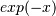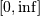numpy.polynomial.laguerre.lagweight¶

numpy.polynomial.laguerre.lagweight(x)[source]

Weight function of the Laguerre polynomials.

The weight function isand the interval of integration is. The Laguerre polynomials are orthogonal, but not normalized, with respect to this weight function.

Parameters : x : array_like Values at which the weight function will be computed. w : ndarray The weight function at x.

Notes

Previous topic

numpy.polynomial.laguerre.laggauss

Next topic

numpy.polynomial.laguerre.lagcompanion